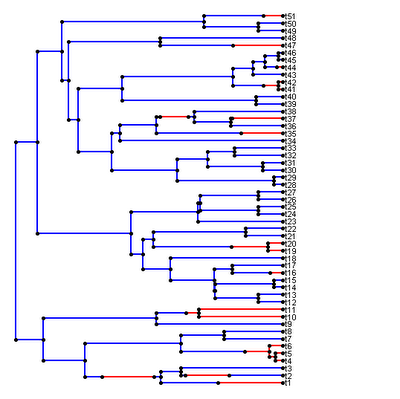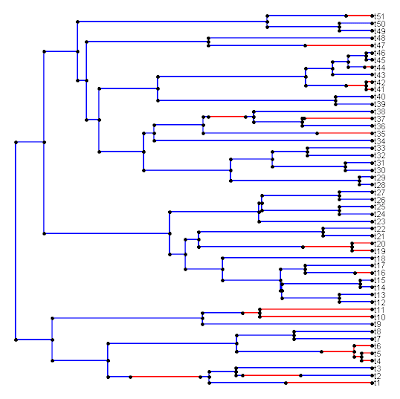## Tuesday, August 16, 2011

### Update to plotSimmap()

In playing around with read.simmap(), I also identified a small issue with the stochastic map plotting function, plotSimmap(). That is, for trees with very short total length (as might be the case with a very slow evolving gene - for instance), the plotted tree is left-aligned in the plotting window and a large amount of white space can be left to right of the tip labels.

To illustrate this, I can first simulate the tree & data:

> require(phytools); require(geiger)
> set.seed(1)
> tree<-rbdtree(b=30,d=0,Tmax=0.1)
> x<-sim.char(tree,model.matrix=list(matrix(c(-10,10,10,-10),2,2)), model="discrete")[,,1]
> mtree<-make.simmap(tree,x)
> cols<-c("blue","red"); names(cols)<-c(1,2)
> plotSimmap(mtree,fsize=0.7,cols)

Which produces the following result:I had the hardest time trying to resolve this issue; however I finally realized that if I first rescaled the sum of the total tree length and the maximum string width of the longest taxon name (which can be found using strwidth(), once a plotting window has been opened) to 1.0, then the problem is solved.

Let's load the new version of plotSimmap() and try again:

> source("http://faculty.umb.edu/liam.revell/phytools/plotSimmap/v0.6/plotSimmap.R")
> plotSimmap(mtree,fsize=0.7,cols)Indeed, this looks much better.

Direct link to code for this function is here. I have only tried this on one system - so I would be happy to hear reports that it works on other machines (particularly Mac OS or Linux).